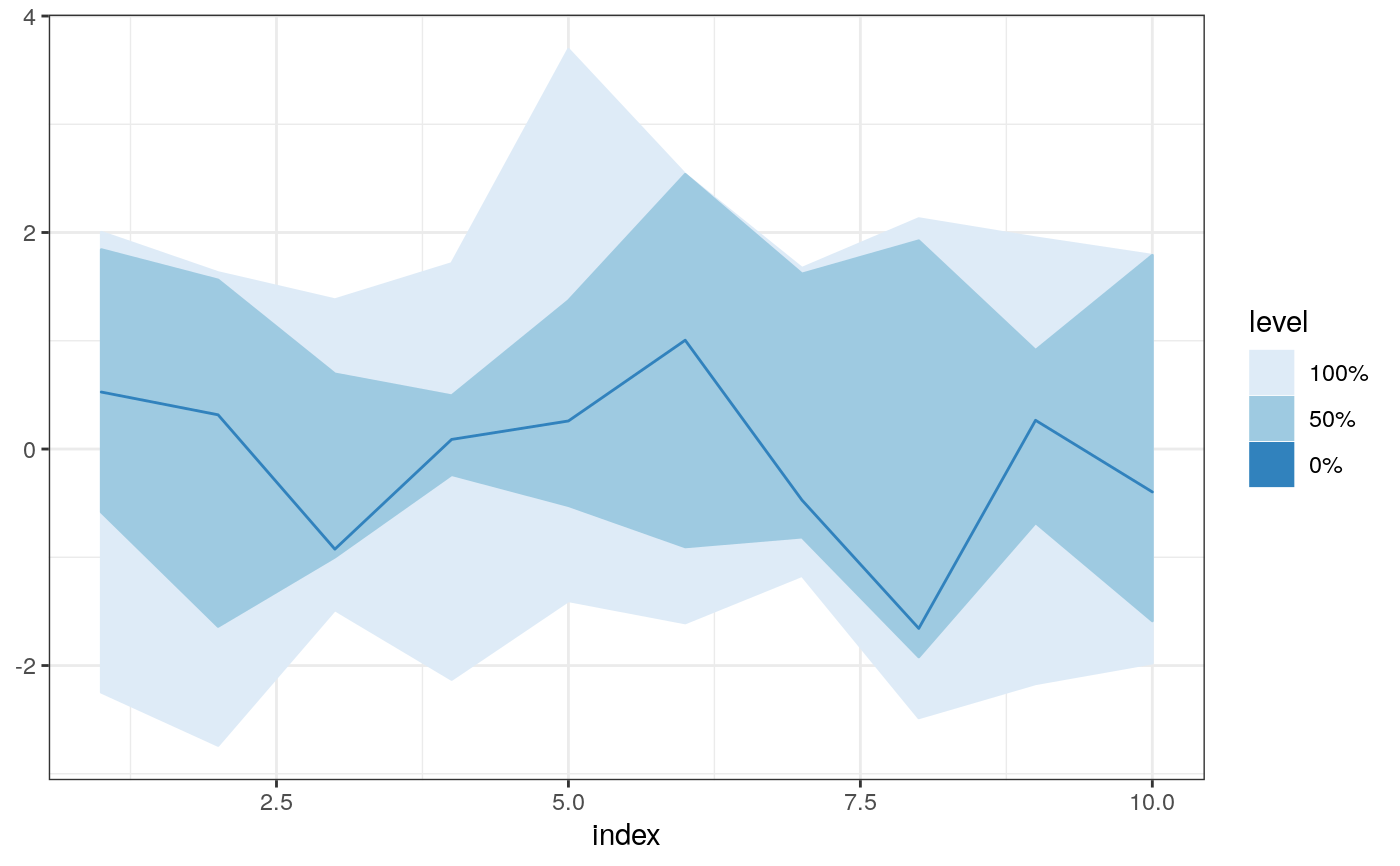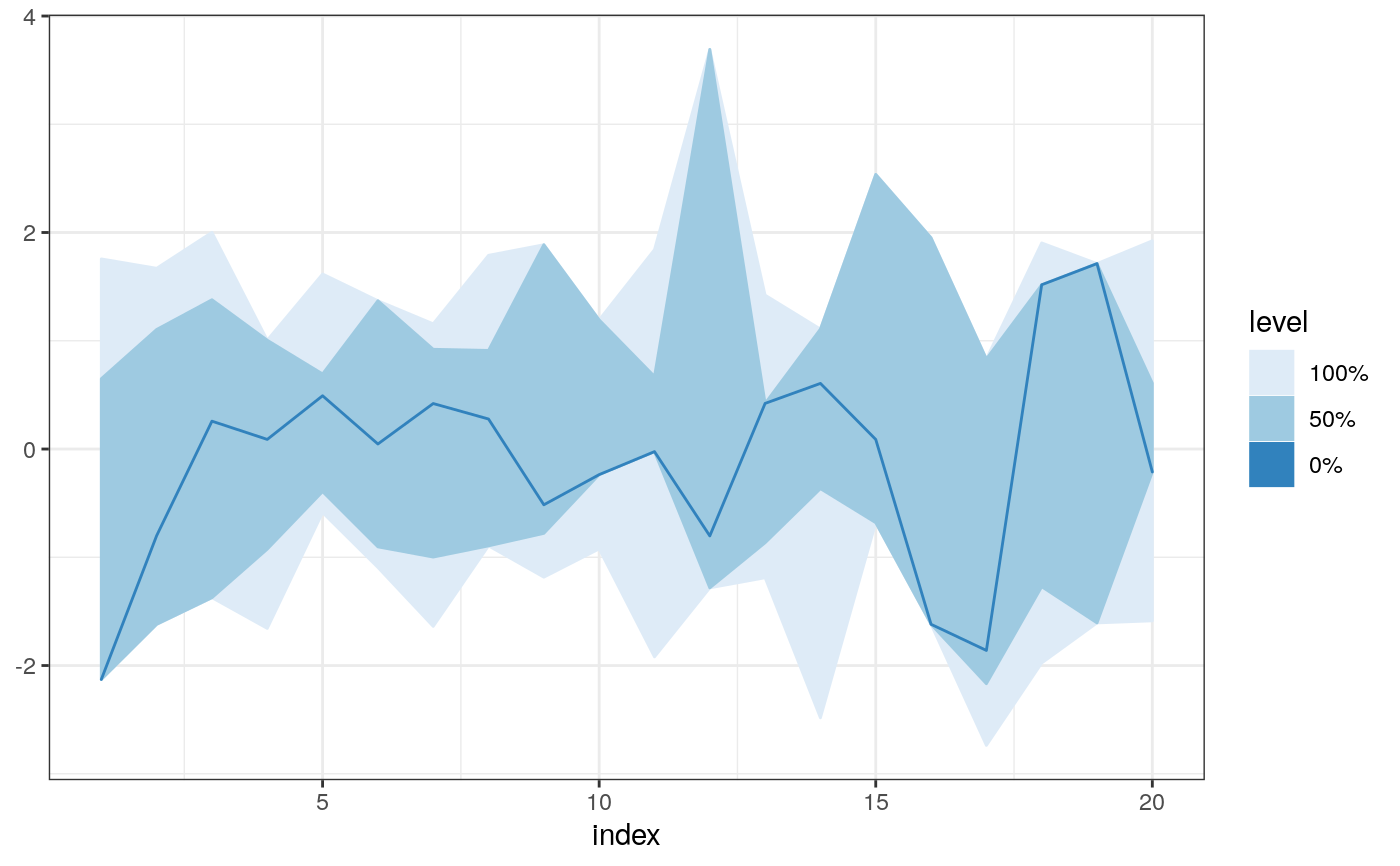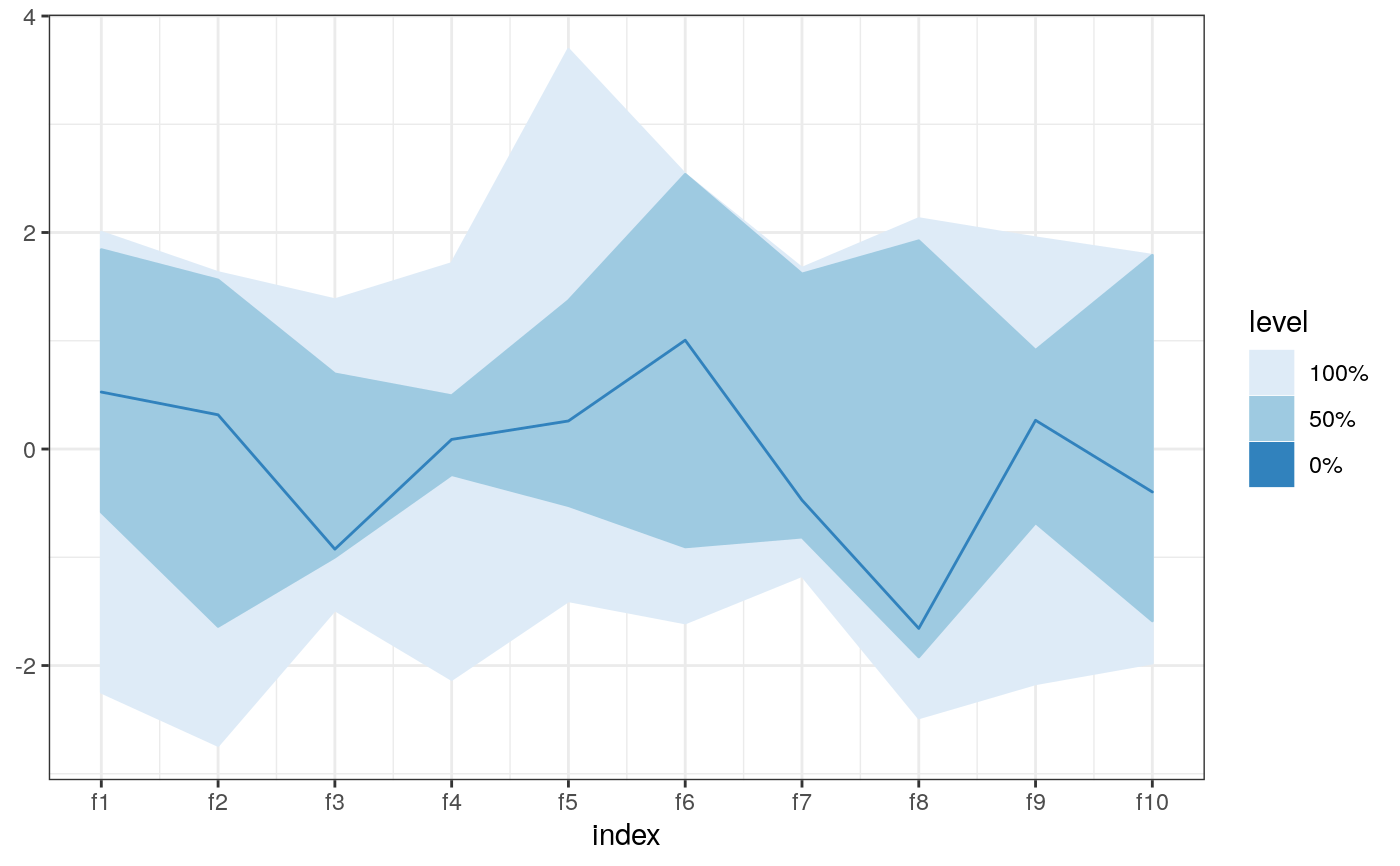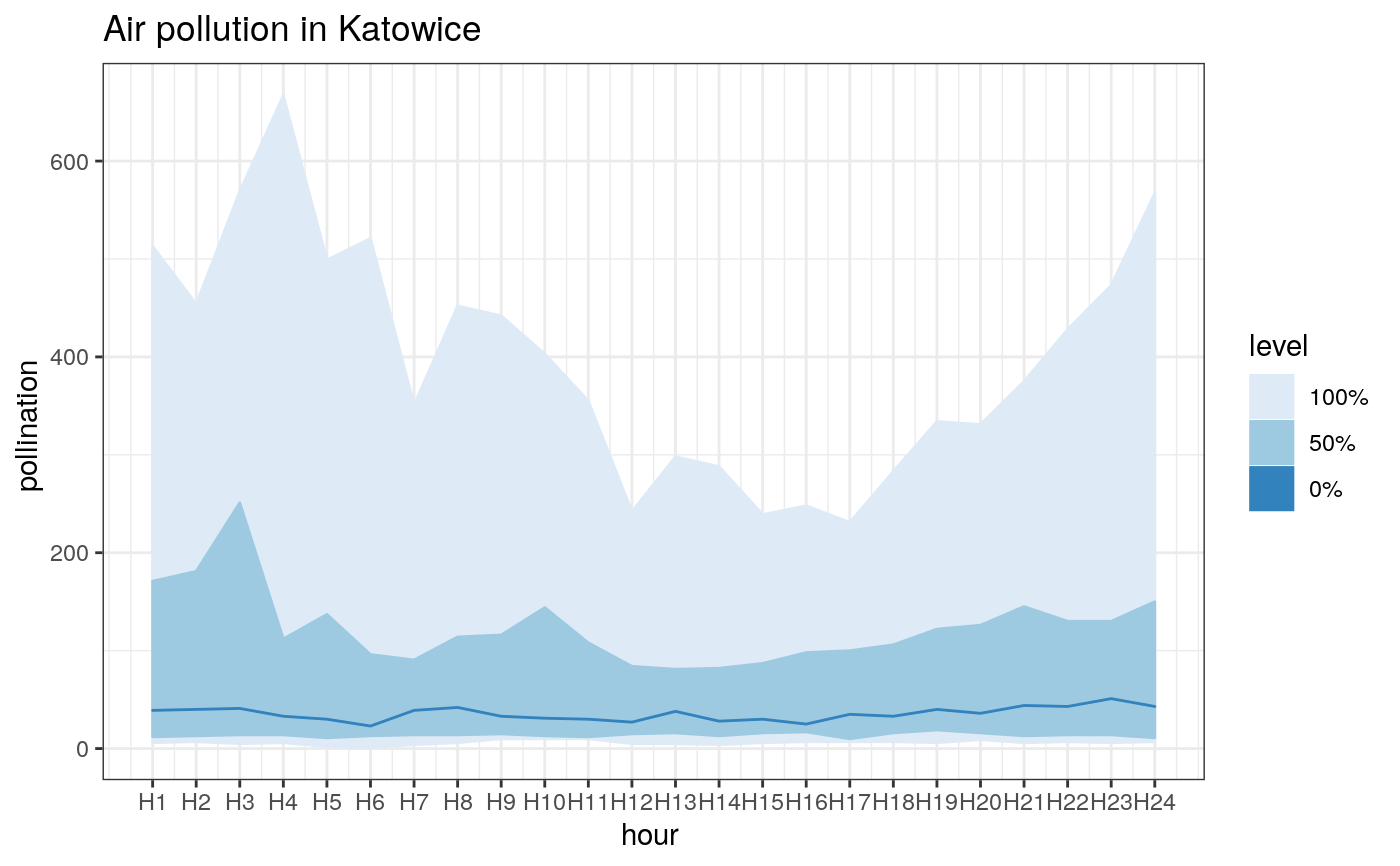Functional boxplot based on Modified Band Depth

fncBoxPlot(u, X = NULL, bands = c(0, 0.5), method = "MBD", byrow = NULL, ...)

## Arguments

u data matrix reference set. If null u will be used as reference. limits for bands depth method byrow other arguments passed to fncDepth

## Examples


# some data:
x <- matrix(rnorm(200), ncol = 10)

fncBoxPlot(x, bands = c(0, 0.5, 1), method = "FM")fncBoxPlot(x, bands = c(0, 0.5, 1), method = "FM", byrow = FALSE)colnames(x) <- paste0("f", 1:ncol(x))
fncBoxPlot(x, bands = c(0, 0.5, 1), method = "FM")# fncBoxPlot handles zoo and xts objects
fncBoxPlot(x_xts, bands = c(0, 0.5, 1), method = "FM")pl + ggtitle("Air pollution in Katowice") + labs(y= "pollination ", x = "hour ")